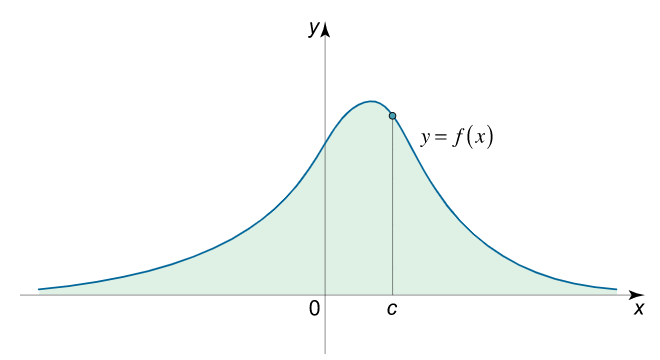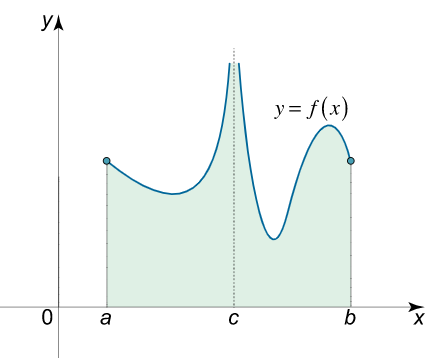Uh Oh! It seems you’re using an Ad blocker!

Since we’ve struggled a lot to makes online calculations for you, we are appealing to you to grant us by disabling the Ad blocker for this domain.# Improper Integral Calculator

Enter a function:W.R.T

Lower Limit: ?

Upper Limit: ?

Table of Content

 1 What is improper integral? 2 Types of improper integrals: 3 How to evaluate the improper integral? 4 How do you know if an integral is improper? 5 Can you spit an improper integral? 6 Is 0 convergent or divergent? 7 What do you mean by convergence in real life?

Get the Widget!

Add this calculator to your site and lets users to perform easy calculations.

Feedback

How easy was it to use our calculator? Did you face any problem, tell us!

An online improper integral calculator is specially designed to measure the integral with defined limits. You can also determine whether the given function is convergent or divergent by using a convergent or divergent integral calculator.

Before we start using this free calculator, let us discuss the basic concept of improper integral.

## What is Improper Integral?

In the context of calculus, an improper integral is a type of integration that determines the area between a curve. This kind of integral has an upper limit and a lower limit. An improper integral can be considered as a type of definite integral. An improper integral is said to be a reversal process of differentiation. Subjecting to an online improper integral calculator is one of the key methods that are best described to solve an improper integral.

### Types of Improper Integrals:

Depending upon the limits we use, there exist two types of improper integral.

Type 1 (Integration Over an Infinite Domain):

In type one, we classify those improper integral that contain upper and lower limits as infinity. We must keep in mind that infinity is a never-ending process and can’t be considered as a number.

Let us suppose that we have a function f(x) which is defined for the interval [a, ∞). Now, if we consider to integrate over a finite domain, the limits become:

$${\int\limits_a^\infty {f\left( x \right)dx} }={ \lim\limits_{n \to \infty } \int\limits_a^n {f\left( x \right)dx} .}$$If the function is defined for the interval(-∞, b], then the integral becomes:

$${\int\limits_{ – \infty }^b {f\left( x \right)dx} }={ \lim\limits_{n \to – \infty } \int\limits_n^b {f\left( x \right)dx} .}$$It should be remembered that if the limits are finite and result in a number, the improper integral is convergent. But if limits are not a number, then the given integral is divergent.

Now, let us discuss the case in which our improper integral has two infinite limits. In this situation, we choose an arbitrary point and break the integral at that particular point. After doing so, we get two integrals having one of the two limits as infinite.

$${\int\limits_{ – \infty }^\infty {f\left( x \right)dx} } = {\int\limits_{ – \infty }^c {f\left( x \right)dx} }+{ \int\limits_c^\infty {f\left( x \right)dx} .}$$These kind of integrals can easily be evaluated with the help of free online improper integral calculator.

Type 2(Improper Integrals With Infinite Discontinuity):

These integrals have undefined integrands at one or more points of integration.
Let f(x) is a function that is discontinuous at x = b and is continuous in the interval [a, b).

$${\int\limits_a^b {f\left( x \right)dx} }={ \lim\limits_{\tau \to 0 + } \int\limits_a^{b – \tau } {f\left( x \right)dx} .}$$Like above, we consider that our function is continuous at the interval (a, b] and discontinuous at x = a.

$${\int\limits_a^b {f\left( x \right)dx} }={ \lim\limits_{\tau \to 0 + } \int\limits_{a + \tau }^b {f\left( x \right)dx} .}$$Now if the function is continuous at the interval (a, c] (c, b] with a discontinuity at x = c.

$${\int\limits_a^b {f\left( x \right)dx} } = {\int\limits_a^c {f\left( x \right)dx} }+{ \int\limits_c^b {f\left( x \right)dx} ,}$$### How to Evaluate the Improper Integral?

We can approach various clever methods to solve an improper integral. Let us solve some examples to understand the concept more clearly.

Example # 01:

Evaluate the improper integral given:

$$\int\limits_{0}^\infty \frac{1}{x} dx$$

Solution:

$$\int\limits_{0}^{\infty} \frac{1}{x}\, dx$$

First, we need to calculate the definite integral:

$$\int \frac{1}{x}\, dx = \log{\left(x \right)}$$   (for steps, see Integral Calculator).

$$\left(\log{\left(x \right)}\right)|_{x=0}=- f i n$$

$$\lim_{x \to \infty}\left(\log{\left(x \right)}\right)=\infty$$

$$\int\limits_{0}^{\infty} \frac{1}{x}\, dx = \left(\left(\log{\left(x \right)}\right)|_{x=0} \right) – \left(\lim_{x \to \infty}\left(\log{\left(x \right)}\right(\right) = \infty$$

$$\int\limits_{0}^{\infty} \frac{1}{x}\, dx=\infty$$

Since the value of the integral is not a finite number, so the integral is divergent. Moreover, the integral convergence calculator is the best option to obtain more precise results.

Example # 02:

Evaluate the improper integral:

$$\int\limits_{-1}^{\infty} \frac{1}{x^{2}}\, dx$$

Solution:

As the given input is:

$$\int\limits_{-1}^{\infty} \frac{1}{x^{2}}\, dx$$

So, we have to solve for the indefinite integral first:

$$\int \frac{1}{x^{2}}\, dx = – \frac{1}{x}$$   (for steps, see Integral Calculator).

$$\left(- \frac{1}{x}\right)|_{x=-1}=1.0$$

$$\lim_{x \to \infty}\left(- \frac{1}{x}\right)=0$$

$$\int\limits_{-1}^{\infty} \frac{1}{x^{2}}\, dx = \left(\left(- \frac{1}{x}\right)|_{x=-1}\right) – \left(\lim_{x \to \infty}\left(- \frac{1}{x}\right)\right) = -1.0$$

$$\int\limits_{-1}^{\infty} \frac{1}{x^{2}}\, dx=-1.0$$

As we have got a finite number, the given integral is said to be convergent. Also, you can clear your doubts by feeding the same function in integral of convergence calculator.

Example # 03:

Evaluate the improper integral given below:

$$\int\limits_{-\infty}^{\infty} \left(2 x^{2} – 2\right)\, dx$$

Solution:

First, we have to determine the indefinite integral:

$$\int \left(2 x^{2} – 2\right)\, dx = \frac{2 x \left(x^{2} – 3\right)}{3}$$   (for steps, see Integral Calculator).

$$\lim_{x \to -∞}\left(\frac{2 x \left(x^{2} – 3\right)}{3}\right)=-\infty$$

$$\lim_{x \to \infty}\left(\frac{2 x \left(x^{2} – 3\right)}{3}\right)=\infty$$

$$\int\limits_{-\infty}^{\infty} \left(2 x^{2} – 2\right)\, dx = \left(\lim_{x \to -∞}\left(\frac{2 x \left(x^{2} – 3\right)}{3}\right)\right) – \left(\lim_{x \to \infty}\left(\frac{2 x \left(x^{2} -3\right)}{3}\right) \right) = \infty$$

$$\int\limits_{-\infty}^{\infty} \left(2 x^{2} – 2\right)\, dx=\infty$$

As the integral is not a finite number so it is said to be divergent. For verification, you can use our free online improper integrals calculator.

### How Improper Integral Calculator Works?

We can adopt different ways to evaluate the improper integral. However, the best option is to use our free divergent or convergent integral calculator.

Let us see what we need to do while using an improper integral calculator.

Input:

• Select the variable w.r.t which you wish to determine the integral
• Select your desired limits for integration
• Click ‘calculate’

Output:

Our improper integral solver calculates:

• The definite or indefinite integral
• Apply limits to determine whether the integral in convergent or divergent
• Shows step-by-step calculations carried out.

## FAQ’s:

### How do you know if an integral is improper?

If an integral has either upper, lower or both limits as infinite, you can say that this is an improper integral.

### Can you split an improper integral?

Yes, splitting an improper integral at 0 is a little bit easier but you can also split it at any number you want.

### Is 0 convergent or divergent?

Whenever you add terms of the sequence that get closer and closer to 0, we can say that the sum is always converging at some finite value. That is why if the terms get small and small enough, we say that the integral does not diverge.

### What do you mean by convergence in real life?

In real life, we should know about the convergence theory, also known as catch-up effect which states that “poorer economies tend to grow at a faster rate than more developed economies”.

## Conclusion:

Determining the area under a curve with the help of an improper integral is a suitable approach as it enables you to understand the period in which the integral gives some value. You can not compute an improper integral using a normal Riemann integral. However, the use of an online improper integral calculator makes it easy to determine whether the given function is convergent or divergent for the limits defined.

## Reference:

From the source of Wikipedia: Convergence of the integral, Types of integrals, Improper Riemann integrals, and Lebesgue integrals, Cauchy principal value, Multivariable improper integrals.

From the source of khan academy: Improper integrals, Divergent improper integral.

Fro the source opf lumen learning: Basic Integration Principles, Properties, Integration By Parts, Trigonometric Integrals, Trigonometric Substitution, The Method of Partial Fractions, Integration Using Tables and Computers, Approximate Integration, Numerical Integration.